https://doi.org/10.5194/acp-18-13155-2018
https://doi.org/10.5194/acp-18-13155-2018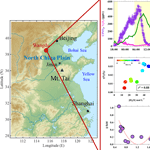# Heterogeneous N2O5 uptake coefficient and production yield of ClNO2 in polluted northern China: roles of aerosol water content and chemical composition

Yee Jun Tham, Zhe Wang, Qinyi Li, Weihao Wang, Xinfeng Wang, Keding Lu, Nan Ma, Chao Yan, Simonas Kecorius, Alfred Wiedensohler, Yuanhang Zhang, and Tao Wang
Abstract

Heterogeneous uptake of dinitrogen pentoxide (N2O5) and production of nitryl chloride (ClNO2) are important nocturnal atmospheric processes that have significant implications for the production of secondary pollutants. However, the understanding of N2O5 uptake processes and ClNO2 production remains limited, especially in China. This study presents a field investigation of the N2O5 heterogeneous uptake coefficient (γ(N2O5)) and ClNO2 production yield (ϕ) in a polluted area of northern China during the summer of 2014. The N2O5 uptake coefficient and ClNO2 yield were estimated by using the simultaneously measured ClNO2 and total nitrate in 10 selected cases, which have concurrent increases in the ClNO2 and nitrate concentrations and relatively stable environmental conditions. The determined γ(N2O5) and ϕ values varied greatly, with an average of 0.022 for γ(N2O5) (±0.012, standard deviation) and 0.34 for ϕ (±0.28, standard deviation). The variations in γ(N2O5) could not be fully explained by the previously derived parameterizations of N2O5 uptake that consider nitrate, chloride, and the organic coating. Heterogeneous uptake of N2O5 was found to have a strong positive dependence on the relative humidity and aerosol water content. This result suggests that the heterogeneous uptake of N2O5 in Wangdu is governed mainly by the amount of water in the aerosol, and is strongly water limited, which is different from most of the field observations in the US and Europe. The ClNO2 yield estimated from the parameterization was also overestimated comparing to that derived from the observation. The observation-derived ϕ showed a decreasing trend with an increasing ratio of acetonitrile to carbon monoxide, an indicator of biomass burning emissions, which suggests a possible suppressive effect on the production yield of ClNO2 in the plumes influenced by biomass burning in this region. The findings of this study illustrate the need to improve our understanding and to parameterize the key factors for γ(N2O5) and ϕ to accurately assess photochemical and haze pollution.

Share
Dates
1 Introduction

The nocturnal heterogeneous reaction of dinitrogen pentoxide (N2O5) with aerosols is a loss pathway of NOx and a source of aerosol nitrate and gas-phase nitryl chloride (ClNO2) (Brown et al., 2006; Osthoff et al., 2008; Thornton et al., 2010; Sarwar et al., 2014) and thereby has important implications for air quality (e.g., Li et al., 2016; Tang et al., 2017). The process begins with the accumulation of gas-phase nitrate radical (NO3) after sunset via the oxidation of nitrogen dioxide (NO2) by O3 and further reaction of NO3 with another NO2, yielding N2O5. The accommodation of N2O5 on the aqueous surface of the aerosol (Reaction R1) and reaction with liquid water (H2O) leads to the formation of a protonated nitric acid intermediate (${\mathrm{H}}_{\mathrm{2}}{\mathrm{ONO}}_{\mathrm{2}}^{+}$) and a nitrate (${\mathrm{NO}}_{\mathrm{3}}^{-}$) (Reaction R2; Thornton and Abbatt, 2005; Bertram and Thornton, 2009).

$\begin{array}{}\text{(R1)}& & {\mathrm{N}}_{\mathrm{2}}{\mathrm{O}}_{\mathrm{5}}\left(\mathrm{g}\right)⟷{\mathrm{N}}_{\mathrm{2}}{\mathrm{O}}_{\mathrm{5}}\left(\mathrm{aq}\right)\text{(R2)}& & {\mathrm{N}}_{\mathrm{2}}{\mathrm{O}}_{\mathrm{5}}\left(\mathrm{aq}\right)+{\mathrm{H}}_{\mathrm{2}}\mathrm{O}\left(\mathrm{l}\right)⟷{\mathrm{NO}}_{\mathrm{3}}\left(\mathrm{aq}\right)+{\mathrm{H}}_{\mathrm{2}}{\mathrm{ONO}}_{\mathrm{2}}^{+}\left(\mathrm{aq}\right)\end{array}$

The ${\mathrm{H}}_{\mathrm{2}}{\mathrm{ONO}}_{\mathrm{2}}^{+}$ will proceed by reacting with another H2O to form an aqueous nitric acid (HNO3; Reaction R3). If chloride (Cl) is present in the aerosols, the ${\mathrm{H}}_{\mathrm{2}}{\mathrm{ONO}}_{\mathrm{2}}^{+}$ will undergo another pathway to produce a nitryl chloride (ClNO2) through Reaction (R4), which is a dominant source of highly reactive chlorine radicals in the troposphere (e.g., Riedel et al., 2012a, 2014).

$\begin{array}{}\text{(R3)}& & {\mathrm{H}}_{\mathrm{2}}{\mathrm{ONO}}_{\mathrm{2}}^{+}\left(\mathrm{aq}\right)+{\mathrm{H}}_{\mathrm{2}}\mathrm{O}\left(\mathrm{l}\right)\to {\mathrm{H}}_{\mathrm{3}}{\mathrm{O}}^{+}\left(\mathrm{aq}\right)+{\mathrm{HNO}}_{\mathrm{3}}\left(\mathrm{aq}\right)\text{(R4)}& & {\mathrm{H}}_{\mathrm{2}}{\mathrm{ONO}}_{\mathrm{2}}^{+}\left(\mathrm{aq}\right)+{\mathrm{Cl}}^{-}\left(\mathrm{aq}\right)\to {\mathrm{ClNO}}_{\mathrm{2}}+{\mathrm{H}}_{\mathrm{2}}\mathrm{O}\left(\mathrm{l}\right)\end{array}$

The heterogeneous loss rate of N2O5 (k(N2O5)het) and the ClNO2 production rate (p(ClNO2)) are fundamentally governed by the probability of N2O5 lost upon collision with particle surface area in a volume of air (i.e., uptake coefficient, γ(N2O5)) and the ClNO2 yield (ϕ), which is defined as the branching ratio between the formation of HNO3 via Reaction (R3) and ClNO2 via Reaction (R4). Assuming that the gas-phase diffusion to the aerosol surfaces is negligible, their relationship can be described by Eqs. (1) and (2), in which ${c}_{{\mathrm{N}}_{\mathrm{2}}{\mathrm{O}}_{\mathrm{5}}}$ is the average molecular speed of N2O5 and Sa is the aerosol surface area.

$\begin{array}{}\text{(1)}& & k\left({\mathrm{N}}_{\mathrm{2}}{\mathrm{O}}_{\mathrm{5}}{\right)}_{\mathrm{het}}=\frac{\mathrm{1}}{\mathrm{4}}{c}_{{\mathrm{N}}_{\mathrm{2}}{\mathrm{O}}_{\mathrm{5}}}\mathit{\gamma }\left({\mathrm{N}}_{\mathrm{2}}{\mathrm{O}}_{\mathrm{5}}\right){S}_{\mathrm{a}}\text{(2)}& & p\left({\mathrm{ClNO}}_{\mathrm{2}}\right)=k\left({\mathrm{N}}_{\mathrm{2}}{\mathrm{O}}_{\mathrm{5}}{\right)}_{\mathrm{het}}\left[{\mathrm{N}}_{\mathrm{2}}{\mathrm{O}}_{\mathrm{5}}\right]\mathit{\varphi }\end{array}$

N2O5 uptake has been shown in the laboratory to be susceptible to changes in the water content, chloride, nitrate, and organic particle coatings in aerosols (e.g., Mentel et al., 1999; Bertram and Thornton, 2009; Tang et al., 2014). The presence of liquid water on the aerosols allows for the accommodation of N2O5 (Reaction R1) and acts as a medium for the solvation process of N2O5 (Reaction R2). It has been found that N2O5 uptake is significantly enhanced in humid conditions than in dry conditions (e.g., Hallquist et al., 2003; Bertram and Thornton, 2009; Gržinić et al., 2015). Higher loading of ${\mathrm{NO}}_{\mathrm{3}}^{-}$ in the aerosol can dramatically decrease N2O5 uptake by reversing the solvation/ionization process of N2O5, shifting the equilibrium in Reaction (R2) to the left to reproduce N2O5, which can be diffused out of the aerosol (known as the “nitrate suppression” effect). The rate of the reversible reaction of Reaction (R2) (i.e., ${\mathrm{H}}_{\mathrm{2}}{\mathrm{ONO}}_{\mathrm{2}}^{+}$ with ${\mathrm{NO}}_{\mathrm{3}}^{-}$) was documented to be 30 to 40 times faster than the reaction of ${\mathrm{H}}_{\mathrm{2}}{\mathrm{ONO}}_{\mathrm{2}}^{+}$ with liquid water in Reaction (R3) (Bertram and Thornton, 2009; Griffiths et al., 2009). The presence of Cl in the aerosol, in contrast, can enhance the reactive uptake because Cl reacts effectively with ${\mathrm{H}}_{\mathrm{2}}{\mathrm{ONO}}_{\mathrm{2}}^{+}$ (in Reaction R4), thus negating the nitrate suppression effect by shifting the equilibrium in Reaction (R2) to the right (Finlayson-Pitts et al., 1989; Bertram and Thornton, 2009). The uptake of N2O5 can also be hindered by the presence of organics, because the organic coating layer on the aerosol could lower the liquid water content and/or limit the surface activity, thus suppressing the accommodation of N2O5 (e.g., Cosman et al., 2008; Gaston et al., 2014).

As for the ClNO2 yield from N2O5 heterogeneous reactions, it was found to be dependent on the fate of ${\mathrm{H}}_{\mathrm{2}}{\mathrm{ONO}}_{\mathrm{2}}^{+}$ and thus on the relative amount of Cl and water content (Benhke et al., 1997; Roberts et al., 2009; Bertram and Thornton, 2009). Therefore, ϕ can be expressed by the following equation (Eq. 3).

$\begin{array}{}\text{(3)}& \mathit{\varphi }\text{param.}=\frac{\mathrm{1}}{\frac{{k}_{\mathrm{R}\mathrm{3}}\left[{\mathrm{H}}_{\mathrm{2}}\mathrm{O}\right]}{{k}_{\mathrm{R}\mathrm{4}}\left[{\mathrm{Cl}}^{-}\right]}+\mathrm{1}}\end{array}$

Roberts et al. (2009) and reference therein reported that the coefficient rate of kR4 is about 450–836 times faster than that of kR3, indicating that ${\mathrm{H}}_{\mathrm{2}}{\mathrm{ONO}}_{\mathrm{2}}^{+}$ proceeds more favorably via Reaction (R4), even with a small amount of Cl. However, some laboratory experiments have suggested that the presence of halides (i.e., bromide), phenols, and humic acid may significantly reduce the ϕ (e.g., Schweitzer et al., 1998; Ryder et al., 2015).

The parameterization of γ(N2O5) and ϕ as a function of the aerosol water content and aerosol chemical composition, derived based on the findings of the laboratory studies mentioned above (e.g., Antilla et al., 2006; Bertram and Thornton, 2009; Davis et al., 2008), have recently been compared with the ambient observations in different environments (Morgan et al., 2015; Phillips et al., 2016; Chang et al., 2016; Z. Wang et al., 2017; McDuffie et al., 2018). Large discrepancies were observed between the γ(N2O5) and ϕ values determined in the field and the laboratory parameterizations derived with pure or mixed aerosol samples, and the differences can be up to 1 order of magnitude. Several reasons have been proposed for the discrepancies between the parameterization and observation values, including the failure of parameterization to account for (1) the complex mixture of organic composition (Bertram et al., 2009; Mielke et al., 2013); (2) the “real” nitrate suppression effect (Riedel et al., 2012b; Morgan et al., 2015); (3) the varying mixing states of the particles (Ryder et al., 2014; X. Wang et al., 2017); and (4) bulk or surface reactions on different particles (e.g., Gaston and Thornton, 2016). These results suggest a lack of comprehensive understanding of the N2O5 uptake and ClNO2 production yield in various atmospheric environments around the world.

Most of the previous field studies of N2O5 uptake and ClNO2 production have been conducted in the United States of America (US) and Europe (Brown et al., 2009; Chang et al., 2016). Direct field investigation of the N2O5 heterogeneous processes in China is very limited. Pathak et al. (2009, 2011) analyzed the aerosol composition and suggested that the accumulation of fine ${\mathrm{NO}}_{\mathrm{3}}^{-}$ aerosol downwind of Beijing and Shanghai was due to significant N2O5 heterogeneous reactions. Wang et al. (2013) linked the observed ${\mathrm{NO}}_{\mathrm{3}}^{-}$ with the precursors of N2O5 (i.e., NO2 and O3) in urban Shanghai and suggested that the N2O5 heterogeneous uptake dominated ${\mathrm{NO}}_{\mathrm{3}}^{-}$ formation on polluted days. However, field measurements of N2O5 and ClNO2 were not available until recently at several sites in southern and northern China (Tham et al., 2014; Z. Wang et al., 2017).

In the summer of 2014, a field campaign was carried out to investigate ClNO2 and N2O5 at a semirural ground site at Wangdu in polluted northern China (Tham et al., 2016). Elevated levels of ClNO2 up to 2070 pptv (1 min average), but relatively low values of N2O5 (1 min average maximum of 430 pptv), were observed on most nights at this site, and heterogeneous processes have been shown to have a significant effect on the following day's radical and ozone production at the site (Tham et al., 2016). Yet, the factors that drive the N2O5 heterogeneous uptake and ClNO2 production yield remain unclear. In this study, we further analyze the dataset to investigate this topic. We first derive values for γ(N2O5) and ϕ from the regression analysis of ClNO2 and the total nitrate (HNO3 and particulate ${\mathrm{NO}}_{\mathrm{3}}^{-}$) dataset and then compare the values obtained in the field with various parameterizations derived from the laboratory studies. With the aid of the aerosol composition and meteorological measurements, we illustrate the factors that drive or influence the variations in γ(N2O5) and ϕ at Wangdu. The values for γ(N2O5) and ϕ obtained here are also compared with field results from the literature to provide an overview of the N2O5ClNO2 heterogeneous process observed in various environments around the world.

2 Methods

The measurement site (38.66 N, 115.204 E) is located at a semirural area in Wangdu county of Hebei province, in the northern part of China. The Wangdu site is situated within agricultural land but is bounded by villages and towns. Beijing (the national capital) is about 170 km to the northeast, Tianjin is about 180 km to the east, Shijiazhuang is about 90 km to the southwest, and Baoding is ∼33 km to the northeast. Dozens of major coal-fired power stations are also located in the region. During the study period, frequent biomass burning activity was observed in the surrounding regions. Analysis of the air masses' back trajectories showed that the sampling site was frequently affected by these surrounding anthropogenic sources. Details on the sampling site and the meteorological conditions during the campaign can be found in Tham et al. (2016).

In this study, N2O5 and ClNO2 were measured with an iodide chemical ionization mass spectrometer (CIMS), with which the N2O5 and ClNO2 were detected as the iodide-cluster ions of I(ClNO2) and I(N2O5), similar to those outlined by Kercher et al. (2009). The detection principles, calibration, and inlet maintenance were described in detail in our previous studies (T. Wang et al., 2016; Tham et al., 2016). The CIMS measurement at the Wangdu site was performed from 20 June to 9 July 2014. A corona discharge ion source setup (for generation of iodide primary ions) was used in the CIMS measurement from 20 to 26 June 2014 with detection limits of 16 pptv for N2O5 and 14 pptv for ClNO2 (3σ; 1 min averaged data) but was replaced by a radioactive ion source from 27 June 2014 until the end of the study with detection limits of 7 pptv for N2O5 and 6 pptv for ClNO2 (3σ; 1 min averaged data). The overall uncertainty in the CIMS measurement was estimated to be ±25 %, with a precision of 3 %.

The present study was supported by other auxiliary measurements of aerosol, trace gases, and meteorological parameters, and the detailed instrumentation for these measurements has been listed in a previous paper (Tham et al., 2016). Trace gases including NO, NO2, O3, SO2, CO, and total odd nitrogen (NOy) were measured with online gas analyzers (Tan et al., 2017). A gas aerosol collector coupled to an ion chromatography system was used to measure the ionic compositions of PM2.5, including ${\mathrm{NO}}_{\mathrm{3}}^{-}$, Cl, ${\mathrm{SO}}_{\mathrm{4}}^{\mathrm{2}-}$, ${\mathrm{NH}}_{\mathrm{4}}^{+}$, and gas-phase HNO3 (Dong et al., 2012). The particle surface area concentrations (Sa) were calculated based on the wet ambient particle number size distribution by assuming spherical particles. In brief, the dry-state particle number size distribution was measured with a mobility particle size spectrometer (covering mobility particle diameters of 4 to 800 nm) and an aerodynamic particle size spectrometer (for aerodynamic particle diameters 0.8 to 10 µm). The wet particle number size distribution as a function of the relative humidity (RH) was calculated from a size-resolved kappa-Köhler function determined from realtime measurement of a high humidity tandem differential mobility analyzer (Hennig et al., 2005; Liu et al., 2014). It should be noted that the major uncertainty in Sa calculation was the assumption and application of κ at different size ranges, leading to an overall uncertainty of ±19 %.

Hydroxyl radicals (OH) were measured with the laser-induced fluorescence technique (Tan et al., 2017). Volatile organic compounds (VOCs) including methane, C2C10 hydrocarbons, formaldehyde, and oxygenated hydrocarbons and acetonitrile (CH3CN) were measured with a cavity ring-down spectrometer, an online gas chromatograph equipped with a mass spectrometer and a flame ionization detector, a Hantzsch fluorimetric monitor, and a proton-transfer-reaction mass spectrometer, respectively (Yuan et al., 2010; Wang et al., 2014). Meteorological data including the wind profile, RH, and temperature were measured with an ultrasonic anemometer and a weather station on a 20 m high tower. Detailed descriptions of these instrumentation and measurement techniques at Wangdu can be found in previous publications (e.g., Y. Wang et al., 2016; Min et al., 2016; Tham et al., 2016; Tan et al., 2017).

3 Results and discussion

## 3.1 Nocturnal heterogeneous N2O5 reaction at Wangdu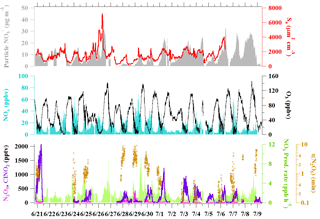Figure 1Time series of concentrations of N2O5, ClNO2, NO3 production rates, the steady-state lifetimes of N2O5, and related gas and aerosol data at Wangdu from 21 June to 9 July 2014. N2O5 and ClNO2 are 1 min averaged data, whereas the NOx, O3, and NO3 production rates and τ(N2O5) are given as 5 min averages. The data for Sa and fine particulate ${\mathrm{NO}}_{\mathrm{3}}^{-}$ are in 10 and 30 min time resolutions, respectively. The data gaps were caused by technical problems, calibrations, or instrument maintenance.

Figure 1 illustrates the time series of NOx, O3, N2O5, ClNO2, particulate ${\mathrm{NO}}_{\mathrm{3}}^{-}$, Sa, the calculated production rate of NO3, and the lifetime of N2O5 observed at Wangdu between 20 June and 9 July 2014. The abundance of NOx and O3 was observed at nighttime (20:00 to 05:00 local time); with average nighttime mixing ratios of 21 and 30 ppbv, respectively. The elevated nighttime NOx and O3 levels led to the active production of NO3, with an average nighttime production rate of NO3 ($={k}_{{\mathrm{O}}_{\mathrm{3}}+{\mathrm{NO}}_{\mathrm{2}}}$[NO2][O3]) of 1.7 ppv h−1 and a maximum level of 8.3 ppv h−1 for the entire campaign. Even with the rapid production of NO3 and the high NO2 level at night, the observed N2O5 concentrations were typically low (i.e., the average nighttime concentration of 34±14 pptv). The low N2O5 value is consistent with the short steady-state lifetime of N2O5 (τ(N2O5)) for the study period, ranging from 0.1 to 10 min, suggesting that the direct loss of N2O5 via heterogeneous reaction and/or indirect loss of N2O5 via decomposition to NO3 (i.e., reactions of NO3 with NO and VOCs) were rapid in this region. The good correlation between nighttime levels of ClNO2 and fine particulate ${\mathrm{NO}}_{\mathrm{3}}^{-}$ (the products of heterogeneous reactions of N2O5 via Reactions R3 and R4, respectively) – with a coefficient of determination (r2) of greater than 0.6 on 10 out of 13 nights (with full CIMS measurement) – provides field evidence of active N2O5 heterogeneous uptake processes in this region.

## 3.2 Estimation of N2O5 uptake coefficient and ClNO2 production yield

The consistent trends and clear correlation between ClNO2 and the ${\mathrm{NO}}_{\mathrm{3}}^{-}$ could be used to quantify N2O5 heterogeneous uptake following the method described by Phillips et al. (2016). The uptake coefficient of N2O5, γ(N2O5), was estimated based on the production rate of ClNO2 (pClNO2) and the nitrate formation rate ($p{\mathrm{NO}}_{\mathrm{3}}^{-}$) from the following Eq. (4). The pClNO2 and $p{\mathrm{NO}}_{\mathrm{3}}^{-}$ were determined from the linear fit of the increase in ClNO2 and total ${\mathrm{NO}}_{\mathrm{3}}^{-}$ (sum of HNO3 and particulate ${\mathrm{NO}}_{\mathrm{3}}^{-}$) with time, while [N2O5] is the mean concentration of N2O5 for the specified duration.

$\begin{array}{}\text{(4)}& \mathit{\gamma }\phantom{\rule{-0.125em}{0ex}}\phantom{\rule{-0.125em}{0ex}}\left({\mathrm{N}}_{\mathrm{2}}{\mathrm{O}}_{\mathrm{5}}\right)=\frac{\mathrm{2}\left(p{\mathrm{ClNO}}_{\mathrm{2}}+p{\mathrm{NO}}_{\mathrm{3}}^{-}\right)}{{C}_{{\mathrm{N}}_{\mathrm{2}}{\mathrm{O}}_{\mathrm{5}}}{S}_{\mathrm{a}}\left[{\mathrm{N}}_{\mathrm{2}}{\mathrm{O}}_{\mathrm{5}}\right]}\end{array}$

The yield of ClNO2 was determined from the regression analysis of ClNO2 versus total ${\mathrm{NO}}_{\mathrm{3}}^{-}$ (Wagner et al., 2012; Riedel et al., 2013). The slope (m) from the regression plot was fitted into Eq. (5) to obtain the ϕ.

$\begin{array}{}\text{(5)}& \mathit{\varphi }=\frac{\mathrm{2}m}{\mathrm{1}+m}\end{array}$

The concentrations of ClNO2, N2O5, total ${\mathrm{NO}}_{\mathrm{3}}^{-}$, and other related data used for this analysis were averaged or interpolated into 10 min. This analysis assumes (1) that the air mass is stable, vertical mixing is limited and losses of ClNO2 and total ${\mathrm{NO}}_{\mathrm{3}}^{-}$ are insignificant within the duration of analysis; and (2) that N2O5 heterogeneous uptake is a dominant source of total soluble nitrate during the night rather than the gas homogeneous production or nitrate production from the preceding daytime.

The limitation of this method is that it cannot predict the γ(N2O5) with negative changes in the concentrations of ClNO2 or total ${\mathrm{NO}}_{\mathrm{3}}^{-}$, which may be a result of differences in the origin or age of the air mass. In accordance with this limitation and with the assumption (1) above, we carefully select plumes during the nighttime that meet the following criterium for the analysis: shorter periods of data, usually between 1.5 and 4 h, with concurrent increases in ClNO2 and total ${\mathrm{NO}}_{\mathrm{3}}^{-}$. The plume age, represented by the ratios of NOx to NOy, was relatively stable (change <0.1 min−1), and no drastic changes were seen in other variables such as wind conditions, the particle surface area, RH, or temperature. Typically, the air masses in the selected cases can be influenced by the emissions from the nearby village or urban area, coal-fired power plants, and biomass burning activities in the region prior to arrival at the site (see Tham et al., 2016). Hence the concentration of NO in the plume must be relatively constant (change of NO∕NO2 ratio <0.1 min−1), as the presence of a transient NO plume may affect the concentration of N2O5, which can bias the estimation of γ(N2O5). Figure 2 shows two examples of relatively constant conditions of relevant chemical composition and environmental variables, together with a plot of ClNO2 versus total ${\mathrm{NO}}_{\mathrm{3}}^{-}$ for the night. Our previous analysis showed that the nighttime vertical mixing is limited at the ground site of Wangdu (Tham et al., 2016), and will likely not affect the analysis of ClNO2 and total ${\mathrm{NO}}_{\mathrm{3}}^{-}$. It should also be noted that contribution of total ${\mathrm{NO}}_{\mathrm{3}}^{-}$ from other sources, like the reaction of OH with NO2 and the oxidation of VOCs by NO3, can bias the values predicted for γ(N2O5) and ϕ.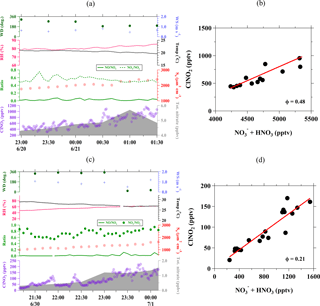Figure 2Example of the accumulation of ClNO2 and total nitrate (particulate ${\mathrm{NO}}_{\mathrm{3}}^{-}$ + HNO3) concentrations during the relatively constant condition of relevant chemical compositions and environmental variables observed for (a) Plume 1 on 20–21 June 2014 and (c) Plume 7 on 29–30 June 2014. Scatter plots of ClNO2 versus particulate ${\mathrm{NO}}_{\mathrm{3}}^{-}$ + HNO3 to estimate the ClNO2 yield (ϕ) for these two cases are shown in (b) and (d).

To check the validity of assumption (2) above, we also calculated the production rate of ${\mathrm{NO}}_{\mathrm{3}}^{-}/{\mathrm{HNO}}_{\mathrm{3}}$ via reaction of OH + NO2 ($={k}_{\mathrm{OH}+{\mathrm{NO}}_{\mathrm{2}}}$[OH][NO2]) and NO3 + VOC (iki[VOCi][NO3], where VOCi=C2H6, C3H6, C3H8, HCHO, CH3OH, C2H4O, CH3C(O)CH3), as shown in the average diurnal profiles of related species in Fig. 3. It is clear that particulate ${\mathrm{NO}}_{\mathrm{3}}^{-}$ was the dominant species during the nighttime at Wangdu, while the nighttime gas-phase HNO3 is only 7 % (on average) of the total ${\mathrm{NO}}_{\mathrm{3}}^{-}$ (Fig. 3b). The strong correlation between ClNO2 and nitrate during the night indicates that the heterogeneous process of N2O5 was the dominant source of particulate nitrate. Moreover, the production rate of HNO3, as calculated from the gas-phase reactions of OH + NO2 and NO3 + VOC, shows a decreasing trend towards the night (Fig. 3c), and the combination of these rates on average is less than one-third of the average $p{\mathrm{NO}}_{\mathrm{3}}^{-}$, which was determined from the slope of nighttime particulate ${\mathrm{NO}}_{\mathrm{3}}^{-}$ in Fig. 3b. The increase in nighttime ${\mathrm{NO}}_{\mathrm{3}}^{-}$ was also accompanied by an increase in ammonium (${\mathrm{NH}}_{\mathrm{4}}^{+}$), which suggests that the repartition process to form ammonium nitrate was efficient, thus limiting the release of HNO3 (Fig. 3d). These results support the validity of the above assumptions and the determination of uptake and yield in this analysis.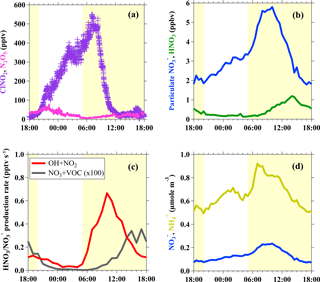Figure 3Diurnal variations in (a) N2O5 and ClNO2; (b) particulate ${\mathrm{NO}}_{\mathrm{3}}^{-}$ and gas-phase HNO3 (c) gas-phase production rate of ${\mathrm{NO}}_{\mathrm{3}}^{-}/{\mathrm{HNO}}_{\mathrm{3}}$ via reaction of OH + NO2 and NO3 + VOC; and (d) concentrations of ${\mathrm{NH}}_{\mathrm{4}}^{+}$ in relation to particulate ${\mathrm{NO}}_{\mathrm{3}}^{-}$. The time indicated in the x axis is the local time and the shaded areas (yellow) represent the daytime.

Table 1N2O5 uptake coefficients and ClNO2 production yields from 10 selected plumes at Wangdu during the summer of 2014. The uncertainty (±) in the γ(N2O5) and ϕ was estimated by the geometric mean of uncertainty from the scattering of the data plots and uncertainty from the measurement of N2O5, ClNO2, aerosol surface area, and total ${\mathrm{NO}}_{\mathrm{3}}^{-}$.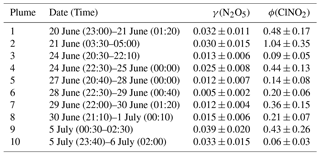With these methods and selection criteria, we can derive γ(N2O5) and ϕ for 10 different nighttime plumes in 8 out of 13 nights with the full CIMS measurement. Table 1 shows the estimated N2O5 uptake coefficients and ClNO2 yields at Wangdu together with the errors that account for the scattering of data in the analysis and uncertainty from the measurement of N2O5, ClNO2, aerosol surface area, and total ${\mathrm{NO}}_{\mathrm{3}}^{-}$. The estimated γ(N2O5) values ranged from 0.005 to 0.039, with a mean value of 0.022. A large variability was found in ϕ (range: 0.06 to 1.04). The relatively larger γ(N2O5) and ϕ values observed on the night of 20–21 June are consistent with the observation of the highest ClNO2 concentration, whereas the lower γ(N2O5) and ϕ values on the night of 28–29 June explain the observation of the elevated N2O5 and small ClNO2 mixing ratios (cf. Fig. 1). The observed γ(N2O5) and ϕ values at Wangdu were compared with literature values derived from previous field observations in various locations in North America, Europe, and China, as summarized in Fig. 4 and Table 2. The variable values of γ(N2O5) in this study fall in the range of γ(N2O5) (<0.001 to 0.11) and ϕ (0.01–1.38) reported around the world. The values are also within the range of N2O5 uptake coefficients and ClNO2 yields determined in regions of China (γ(N2O5) =0.004–0.103; ϕ=0.01–0.98), which are in the middle to upper end of the values reported around the world. The observed significant ClNO2 concentrations and high yields of ϕ here, consistent with other studies at inland sites (cf. Table 2), also point to the fact that ClNO2 production can be efficient in regions far from the oceanic source of chloride and further highlight the important role of anthropogenic chloride emissions in the chlorine activation process and the next day's photochemistry. The question that arises here is what drives the large variability in the γ(N2O5) and ϕ at Wangdu.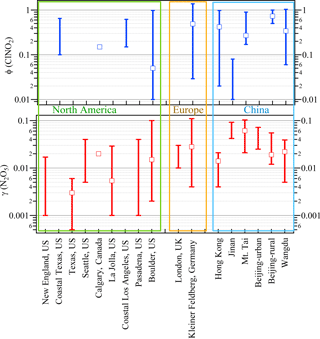Figure 4Comparison of the field-observed N2O5 uptake coefficient and ClNO2 yield from previous studies. Stick bars represent the range of the reported values, and cube represent the median or average values reported in these measurements. The corresponding references are listed in Table 2.

Table 2Summary of field-observed N2O5 uptake coefficient and ClNO2 yield from previous studies.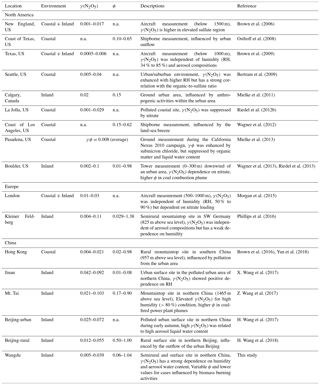n.a. = no information available

## 3.3 Factors that control the N2O5 uptake coefficient

Heterogeneous uptake of N2O5 is governed by various factors, including the amount of water and the physical and chemical characteristics of the aerosols (Chang et al., 2011; Brown and Stutz, 2012). To gain better insight into the factors that drive the N2O5 heterogeneous uptake, the determined γ(N2O5) values were compared with those predicted from complex laboratory-derived parameterizations, and their relationships with the aerosol water content and aerosol compositions observed at Wangdu were examined. The parameterization of N2O5 uptake coefficient derived from Bertram and Thornton (2009; γB&T) assumed a volume-limited reaction of N2O5 on mixed aerosols and considered the bulk amount of nitrate, chloride, and water in the aerosol as the controlling factors, which can be expressed by Eq. (6):

$\begin{array}{}\text{(6)}& {\mathit{\gamma }}_{\mathrm{B}\mathrm{&}\mathrm{T}\phantom{\rule{0.125em}{0ex}}}=Ak\left(\mathrm{1}\phantom{\rule{0.125em}{0ex}}-\frac{\mathrm{1}}{\left(\frac{{k}_{\mathrm{R}\mathrm{3}}\left[{\mathrm{H}}_{\mathrm{2}}\mathrm{O}\right]\left(\mathrm{l}\right)}{{k}_{\mathrm{R}\mathrm{2}\mathrm{b}}\left[{\mathrm{NO}}_{\mathrm{3}}^{-}\right]}\right)+\mathrm{1}+\left(\frac{{k}_{\mathrm{R}\mathrm{4}}\left[{\mathrm{Cl}}^{-}\right]}{{k}_{\mathrm{R}\mathrm{2}\mathrm{b}}\left[{\mathrm{NO}}_{\mathrm{3}}^{-}\right]}\right)}\right),\end{array}$

where A is an empirical pre-factor calculated from the volume of aerosol (V), Sa, ${c}_{{\mathrm{N}}_{\mathrm{2}}{\mathrm{O}}_{\mathrm{5}}}$, and Henry's law coefficient of N2O5 ($A=\mathrm{4}/{c}_{{\mathrm{N}}_{\mathrm{2}}{\mathrm{O}}_{\mathrm{5}}}×V/{S}_{\mathrm{a}}×{H}_{\mathrm{aq}}\right)$; $k=\mathrm{1.15}×{\mathrm{10}}^{\mathrm{6}}-\left(\mathrm{1.15}×{\mathrm{10}}^{\mathrm{6}}{\right)}^{\mathrm{exp}\left(-\mathrm{0.13}\left[{\mathrm{H}}_{\mathrm{2}}\mathrm{O}\right]\right)}$; ${k}_{\mathrm{R}\mathrm{3}}/{k}_{\mathrm{R}\mathrm{2}\mathrm{b}}=\mathrm{0.06}$; and ${k}_{\mathrm{R}\mathrm{4}}/{k}_{\mathrm{R}\mathrm{2}\mathrm{b}}=\mathrm{29}$. The concentration of aerosol liquid water ([H2O](l)) used in this study was estimated from the Extended Aerosol Inorganics Model (E-AIM) model IV with inputs of measured bulk aerosol composition of ${\mathrm{NH}}_{\mathrm{4}}^{+}$, Na+, ${\mathrm{SO}}_{\mathrm{4}}^{\mathrm{2}-}$, ${\mathrm{NO}}_{\mathrm{3}}^{-}$, and Cl (http://www.aim.env.uea.ac.uk /aim/model4/model4a.php, last access: 16 August 2018; Wexler and Clegg, 2002), and the VSa was taken from the field measurement at Wangdu. It should be noted that the parameterization and calculation here assumes an internal mixing of the aerosol chemical species, and the size distribution of [H2O], [${\mathrm{NO}}_{\mathrm{3}}^{-}$], and [Cl] in aerosols was not considered due to lack of measurement information. The uptake process would vary with size and mixing state of the particles, thus the predicted γ values here may be biased as a result, but represents an average over bulk aerosols. The γB&T also does not account for the suppression of γ(N2O5) from the organics, but it is frequently used with the parameterization formulated by Anttila et al. (2006), who treated the organic fraction in the aerosols as a coating, as given in Eq. (7) (e.g., Morgan et al., 2015; Phillips et al., 2016; Chang et al., 2016). The net uptake of N2O5 onto an aqueous core and organic coating (γB&T+Org) can be determined by Eq. (8).

$\begin{array}{}\text{(7)}& & {\mathit{\gamma }}_{\mathrm{Org}}=\frac{\mathrm{4}RT{H}_{\mathrm{org}}{D}_{\mathrm{org}}{R}_{\mathrm{c}}}{{C}_{{\mathrm{N}}_{\mathrm{2}}{\mathrm{O}}_{\mathrm{5}}}L{R}_{\mathrm{p}}}\text{(8)}& & \frac{\mathrm{1}}{{\mathit{\gamma }}_{\mathrm{B}\mathrm{&}\mathrm{T}+\mathrm{Org}}}=\frac{\mathrm{1}}{{\mathit{\gamma }}_{\mathrm{B}\mathrm{&}\mathrm{T}}}+\frac{\mathrm{1}}{{\mathit{\gamma }}_{\mathrm{Org}}}\end{array}$

Here, the Horg is the Henry's law constant of N2O5 for organic coating; Dorg is the solubility and diffusivity of N2O5 in the organic coating of thickness L; and Rc and Rp are the radii of the aqueous core and particle, respectively. The particle radius Rp was determined from the measured median radius of the particle surface area distribution. The L was calculated from the volume ratio of the inorganics to total particle volume following the method in Riemer et al. (2009) with the assumption of a hydrophobic organic coating (density, 1.27 g cm−3) on the aqueous inorganic core (with a density of 1.77 g cm−3). The aqueous core radius Rc was calculated by subtracting the L from Rp. The HorgDorg is equal to 0.03×HaqDaq, where Haq=5000 M atm−1 and ${D}_{\mathrm{aq}}={\mathrm{10}}^{-\mathrm{9}}$ m2 s−1 (Chang et al., 2011, and references therein). In addition, Evans and Jacob (2005) proposed a simpler parameterization of N2O5 uptake on sulfate aerosol (γE&J) as a function of temperature and RH, as given by Eq. (9).

$\begin{array}{ll}& {\mathit{\gamma }}_{\mathrm{E}\mathrm{&}\mathrm{J}}=\left(\mathrm{2.79}×{\mathrm{10}}^{-\mathrm{4}}+\mathrm{1.3}×{\mathrm{10}}^{-\mathrm{4}}×\mathrm{RH}-\mathrm{3.43}×{\mathrm{10}}^{-\mathrm{6}}\\ \text{(9)}& & \phantom{\rule{1em}{0ex}}×{\mathrm{RH}}^{\mathrm{2}}+\mathrm{7.52}×{\mathrm{10}}^{-\mathrm{8}}×{\mathrm{RH}}^{\mathrm{3}}\right)×{\mathrm{10}}^{\left(\mathrm{4}×{\mathrm{10}}^{-\mathrm{2}}×\left(T-\mathrm{294}\right)\right)}\end{array}$

Figure 5 illustrates a comparison of field-derived N2O5 uptake coefficients with the values computed from the above parameterizations. The computed γB&T (red circle) ranged from 0.046 to 0.094 and was consistently higher than the field-derived γ(N2O5) by up to a factor of 9. By accounting for the effects of organic coating on the N2O5 uptake coefficient via Eqs. (7) and (8), the calculated N2O5 uptake coefficients (green circle in Fig. 5) are significantly underestimated. Note that only six cases were available to compute the γB&T+Org due to the limited organic aerosols data for the study period. The N2O5 uptake coefficients computed from the parameterization suggested by Evans and Jacob (2005) are generally consistent with the field-derived γ(N2O5) (as shown by blue circles). The different results from these parameterizations may suggest more complex aerosol composition, mixing states, and other physicochemical properties in the real ambient atmosphere than in the aerosol sample used in the laboratory study.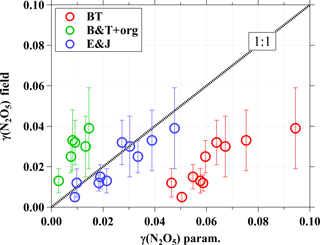Figure 5Comparison of field-derived N2O5 uptake coefficients with values computed from different parameterizations. The dashed line represents 1:1, and the error bars show the uncertainty in γ(N2O5) derived from the field.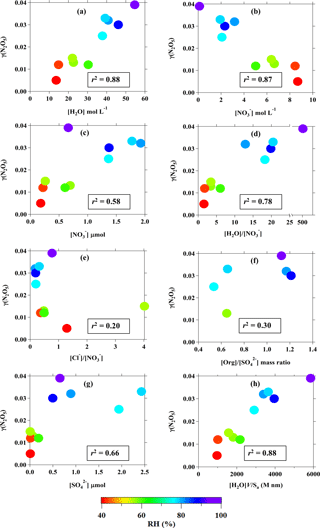Figure 6Relationship between field-derived γ(N2O5) and (a) aerosol water content (mol per volume of aerosol); (b) nitrate concentration per volume of aerosol; (c) particulate nitrate concentration (µmol m−3 of air); (d) H2O to ${\mathrm{NO}}_{\mathrm{3}}^{-}$ molar ratio; (e) Cl to ${\mathrm{NO}}_{\mathrm{3}}^{-}$ molar ratio; (f) organic-to-sulfate mass ratio (data from aerosol mass spectrometer); (g) concentration of ${\mathrm{SO}}_{\mathrm{4}}^{\mathrm{2}-}$ (µmol m−3 of air); and (h) amount of water in aerosol multiplied by the volume-to-surface-area ratio. Color code represents the ambient RH, and the value in the box is the best correlation coefficient obtained from curve fittings.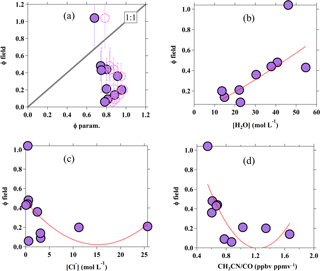Figure 7Scatter plots for (a) yield derived from the field versus yield calculated from the parameterization, using kR4kR3 of 483 (recommended by Bertram and Thornton, 2009; solid circle) and 836 (recommended by Behnke et al., 1997; pink open circle). Error bars represent the uncertainty in field-derived ϕ, and the black dotted line represents the 1:1 ratio; (b) field-derived yield versus aerosol water content; (c) field-derived yield versus chloride; and (d) field-derived yield versus CH3CN∕CO. The red dotted lines show the quadratic fitting line of the data.

We then examined the relationships of the field-derived γ(N2O5) with RH, water content, and aerosol compositions, as illustrated in Fig. 6. It can be seen in Fig. 6a that the γ(N2O5) has a clear correlation with the aerosol water content (r2=0.88; p<0.01, t test). The strong dependence of γ(N2O5) on the aerosol water content was observed for RH ranging from 40 % to exceed 90 % or [H2O] from 10 mol L−1 to above 40 mol L−1. This pattern is different to the trends observed in laboratory studies for N2O5 uptake onto aqueous sulfate and malonic acid aerosols, in which the γ(N2O5) strongly increases with humidity at RH below 40–50 % but becomes insensitive above this threshold (e.g., Hallquist et al., 2003; Thornton et al., 2003). The γ(N2O5) at Wangdu shows a decreasing trend with the concentration of ${\mathrm{NO}}_{\mathrm{3}}^{-}$ per volume of aerosol (see Fig. 6b), which is similar to the results from the previous laboratory studies (Bertram and Thornton, 2009; Griffiths et al., 2009). However, we do not think that [${\mathrm{NO}}_{\mathrm{3}}^{-}$] is the dominant limiting factor for N2O5 uptake at this site – as seen in the consistency of the γ(N2O5) data points with the change in RH (in the color code of Fig. 6b), the increasing trend of γ(N2O5) with the concentration of particulate nitrate in the air (cf. Fig. 6c), and the positive dependency of γ(N2O5) on the molar ratio of [H2O]  [${\mathrm{NO}}_{\mathrm{3}}^{-}$] (cf. Fig. 6d) – which reflect that the N2O5 uptake is more sensitive to the aerosol water content than to the ${\mathrm{NO}}_{\mathrm{3}}^{-}$, at least up to a [H2O] : [${\mathrm{NO}}_{\mathrm{3}}^{-}$] ratio of 20. The increase in ambient particulate nitrate is probably due to the faster N2O5 heterogeneous reaction. The N2O5 uptake does not show an increasing trend with the chloride-to-nitrate molar ratio, a pattern demonstrated in the laboratory result (Bertram and Thornton, 2009), but rather a decrease for high [Cl]  [${\mathrm{NO}}_{\mathrm{3}}^{-}$] ratios, and it also correlates with differences in RH (cf. Fig. 6e). There is a lack of correlation of γ(N2O5) with the [Org] : [${\mathrm{SO}}_{\mathrm{4}}^{\mathrm{2}-}$] ratio observed in the ratio range of 0.5–1.2, indicating that the suppression of organics on the N2O5 uptake may be insignificant at Wangdu. These results are in line with the parameterization comparison results shown in Fig. 5, which reveal that the variation in the N2O5 uptake at Wangdu is not driven by the chemical properties of aerosols like ${\mathrm{NO}}_{\mathrm{3}}^{-}$, Cl, and organics but rather that the RH or the aerosol water content plays a defining role in the N2O5 heterogeneous uptake.

The response of N2O5 uptake to the changes in RH is consistent with the changes in the sulfate (${\mathrm{SO}}_{\mathrm{4}}^{\mathrm{2}-}$) concentrations (see Fig. 6g), which mainly determine the hygroscopicity of the aerosols and were found to be responsible for the particle growth at Wangdu (Wu et al., 2017). The hygroscopic growth of aerosols inferred by the RH (water uptake) can affect the amount of water in the aerosol and the volume-to-surface-area ratio ([H2O]VSa). The good positive correlation of γ(N2O5) with [H2O]VSa (see Fig. 6h) suggests that the increased volume of aerosol, in particular the layer of aerosol water content, could allow efficient diffusion of N2O5 and solvation of N2O5 into ${\mathrm{H}}_{\mathrm{2}}{\mathrm{ONO}}_{\mathrm{2}}^{+}$ and ${\mathrm{NO}}_{\mathrm{3}}^{-}$ for further aqueous reactions, whereas a smaller volume of aerosol (less water content) may be easily saturated by N2O5 and then diffuse the N2O5 out of the aerosol, limiting the solvation of the N2O5 process and restricting N2O5 uptake. These results are consistent with several laboratory studies which have demonstrated that an increase in RH enhanced the particle aqueous volume and increased the bulk reactive N2O5 uptake on aqueous sulfate and organic acids (e.g., malonic, succinic, and glutaric acids) containing aerosols (Thornton et al., 2003; Hallquist et al., 2003). The increase in RH can also lower the viscosity of the aqueous layer in organic-containing aerosols, leading to greater diffusivity of N2O5 within the aerosol water layer, which ultimately increases the N2O5 uptake (Gržinić et al., 2015).

The strong dependency of N2O5 uptake upon the RH has not been clearly demonstrated in other field measurements. Field observations of γ(N2O5) in North America and Europe did not show any significant direct dependence of γ(N2O5) on RH but were strongly influenced by the aerosol composition (refer to the descriptions in Table 2). For instance, the flight measurements in Texas and London showed the independence of γ(N2O5) at RH from 34 % to 90 %, but the γ(N2O5) were generally controlled by the amount of ${\mathrm{NO}}_{\mathrm{3}}^{-}$ and/or organic compounds (Brown et al., 2009; Morgan et al., 2015). A comparison of ground measurements in Seattle and Boulder showed variations in γ(N2O5) at various H2O(l) levels in the two places, but the RH alone was insufficient to describe their observed γ(N2O5) variability, and the organic composition of the aerosols was determined to have a dominant influence on γ(N2O5) (Bertram et al., 2009). Another study from a mountainous site in Germany reported no significant correlation of γ(N2O5) with aerosol compositions and only a weak dependence on humidity (Phillips et al., 2016). However, field measurements at Jinan and Mt. Tai in northern China during the same season also showed a positive relationship of γ(N2O5) with an RH between 43 % and 72 % and aerosol water content of 31–65 mol L−1, respectively (X. Wang et al., 2017; Z. Wang et al., 2017). The results of this study and previously reported results in the region may suggest that RH and aerosol water content are the important limiting factors for the N2O5 heterogeneous process in polluted northern China. In summary, the more complex parameterizations considering nitrate, chloride, and the organic coating cannot fully represent the variation in γ(N2O5) at Wangdu; instead, a simple parameterization that accounts only for temperature and RH appears to explain the variation in γ(N2O5) at Wangdu. It would be of great interest to determine whether such a phenomenon can be found in other places.

## 3.4 Factors that affect the ClNO2 production yield

In addition to the uptake coefficients, the factors that influence the branching yield of ClNO2 from the N2O5 heterogeneous uptake were also assessed. Figure 7a shows the scatter plot of the ϕ calculated from Eq. (3) versus the ClNO2 yield derived from the Wangdu field data from Eq. (5) in Sect. 3.2. Generally, the ϕparam. shows less variability but was obviously overestimated relative to the field-determined ϕ. Such a discrepancy has also been observed elsewhere (Thornton et al., 2010; Mielke et al., 2013; Riedel et al., 2013), including our recent observations in an urban site (Jinan) and a mountaintop site (Mt. Tai) in northern China, where the parameterized ϕ would be overestimated by up to 2 orders of magnitude (X. Wang et al., 2017; Z. Wang et al., 2017). Further analysis by linking the field-derived ClNO2 yields with the aerosol water content (Fig. 7b) and the Cl content (Fig. 7c) show a weak positive correlation (r2=0.36) and a weak negative trend (r2=0.20), respectively (from quadratic fitting). The weak correlations reflect that the ClNO2 yield is not solely controlled by the amount of water and chloride in the aerosol, as defined in the parameterization (see Eq. 3), and/or the existence of other nucleophiles that can compete with Cl in Reactions (R3) and (R4). The aqueous concentration of Cl in the present study is relatively higher than previous laboratory studies (e.g., Bertram and Thornton, 2009; Roberts et al., 2009), and might not be fully involved in the Reaction (R4); for example, the possible effect of the nonuniform distribution of chloride within the aerosol. It might contribute to the overestimation and less variability in ϕ predicted from the parameterization (Riedel et al., 2013) and the positive relationship of field-derived ϕ with [H2O] (see Fig. 7b) might also imply that the increase in water content could increase the availability of the aerosol Cl, thus prompting the Reaction (R4) to increase the ClNO2 production yield.

An interesting observation from Wangdu is that the field-derived ϕ shows a decreasing trend (r2=0.58 from quadratic data fitting) with the ratio of acetonitrile to carbon monoxide (CH3CN∕CO), which is an indicator of biomass burning emission (Christian et al., 2003; Akagi et al., 2011), as illustrated in Fig. 7d. The ϕ decreased at larger CH3CN∕CO ratios, which corresponds to higher [Cl] concentrations per volume of aerosol (cf. Fig. 7c) because biomass burning emits a significant level of chloride particles. The observations here may suggest that the ϕ is likely “suppressed” in air masses influenced by biomass burning, which were frequently observed during the study period (Tham et al., 2016), and is consistent with the recent field observation of much lower concentrations of ClNO2 during the bonfire event in Manchester compared to that after the event (Reyes-Villegas et al., 2017) and with a laboratory experiment which demonstrated that only a small amount (∼10 %) of reacted N2O5 was converted to ClNO2 on the biomass-burning aerosols (Ahern et al., 2018). Another laboratory study showed that the ClNO2 yield can be reduced by as much as 80 % in the presence of aromatic organic compounds like phenol and humic acid in the aerosol (Ryder et al., 2015), and previous studies in China reported abundant humic-like substances (e.g., aromatic organic compounds) in aerosols with a large contribution from biomass burning (Fu et al., 2008). Therefore, the frequently observed influence of biomass burning at the Wangdu site during the campaign could in part explain the lower ϕ values and the discrepancy between observation and parameterization. More studies are needed to investigate the effects of biomass burning emissions on the heterogeneous process.

4 Summary and conclusions

We present an in-depth analysis of the N2O5 uptake coefficient and ClNO2 yield in a polluted northern China environment during the summer of 2014. Large variations in the levels of γ(N2O5) and ϕ were observed during the study, ranging from 0.005 to 0.039 and from 0.06 to 1.04, respectively. A comparison between the γ(N2O5) values derived from the field and the parameterizations that considered the nitrate and chloride levels and the hydrophobic organic coating showed poor agreement, suggesting more complex influences of ambient aerosol properties at the site than with the pure or mixed samples used in the laboratory. The γ(N2O5) values at Wangdu were found to have a clear dependence on the RH and the aerosol water content, a phenomenon that was found in laboratory experiments but has not been observed in previous field studies in the US or Europe. The parameterization that explicitly considers the dependence on RH showed better agreement with the field-derived γ(N2O5) compared to the more complex formulation that considers the aerosol composition. The ClNO2 yield estimated from the parameterization is generally overestimated when compared to the field-derived values. The observed ϕ was found to be suppressed in the air masses influenced by biomass burning even though abundant aerosol chloride was present. The results of this study point to the need for more field and laboratory studies to obtain realistic parameterization of the heterogeneous processes of N2O5 and ClNO2 to better simulate the ozone and aerosol production in air quality models in regions of China with high NOx emissions.

Data availability

The data used in this study are available upon request from the corresponding author (z.wang@polyu.edu.hk).

Author contributions

TW and ZW designed the research in this study; KL and YZ organized the field campaign; YJT, ZW, and WW performed the field measurement; YJT, ZW, and QL analyzed the data; YJT, ZW, and TW wrote the manuscript. Other authors contributed to measurements, discussion, and commented on the manuscript.

Competing interests

The authors declare that they have no conflict of interest.

Special issue statement

Acknowledgements

The authors thank Steven Poon, Qiaozhi Zha, Zheng Xu, and Hao Wang for the logistics support; Liming Cao and Lingyan He for providing the aerosol mass spectrometer data; and to Li Zhang for scientific discussion. This work was funded by the National Natural Science Foundation of China (91544213, 41505103 and 41275123), National Key Research and Development Program of China (2016YFC0200500), Research Grants Council of Hong Kong (15265516), PolyU Project of Strategic Importance (1-ZE13), and the Research Institute for Sustainable Urban Development (RISUD). The Peking University team acknowledges support from the National Natural Science Foundation of China (21190052) and the Strategic Priority Research Program of the Chinese Academy of Sciences (XDB05010500). The Leibniz Institute for Tropospheric Research team acknowledges funding from the Sino German Center (no. GZ663).

Edited by: Luisa Molina
Reviewed by: two anonymous referees

References

Ahern, A., Goldberger, L., Jahl, L., Thornton, J., and Sullivan, R. C.: Production of N2O5 and ClNO2 through nocturnal processing of biomass-burning aerosol, Environ. Sci. Technol., 52, 550–559, https://doi.org/10.1021/acs.est.7b04386, 2017.

Akagi, S. K., Yokelson, R. J., Wiedinmyer, C., Alvarado, M. J., Reid, J. S., Karl, T., Crounse, J. D., and Wennberg, P. O.: Emission factors for open and domestic biomass burning for use in atmospheric models, Atmos. Chem. Phys., 11, 4039–4072, https://doi.org/10.5194/acp-11-4039-2011, 2011.

Anttila, T., Kiendler-Scharr, A., Tillmann, R., and Mentel, T. F.: On the reactive uptake of gaseous compounds by organic-coated aqueous aerosols: theoretical analysis and application to the heterogeneous hydrolysis of N2O5, J. Phys. Chem. A, 110, 10435–10443, https://doi.org/10.1021/jp062403c, 2006.

Behnke, W., George, C., Scheer, V., and Zetzsch, C.: Production and decay of ClNO2, from the reaction of gaseous N2O5 with NaCl solution: Bulk and aerosol experiments, J. Geophys. Res.-Atmos., 102, 3795–3804, https://doi.org/10.1029/96jd03057, 1997.

Bertram, T. H. and Thornton, J. A.: Toward a general parameterization of N2O5 reactivity on aqueous particles: the competing effects of particle liquid water, nitrate and chloride, Atmos. Chem. Phys., 9, 8351–8363, https://doi.org/10.5194/acp-9-8351-2009, 2009.

Bertram, T. H., Thornton, J. A., Riedel, T. P., Middlebrook, A. M., Bahreini, R., Bates, T. S., Quinn, P. K., and Coffman, D. J.: Direct observations of N2O5 reactivity on ambient aerosol particles, Geophys. Res. Lett., 36, L19803, https://doi.org/10.1029/2009gl040248, 2009.

Brown, S., Ryerson, T., Wollny, A., Brock, C., Peltier, R., Sullivan, A., Weber, R., Dube, W., Trainer, M., Meagher, J. Fehsenfeld, F. C., and Ravishankara, A. R.: Variability in nocturnal nitrogen oxide processing and its role in regional air quality, Science, 311, 67–70, 2006.

Brown, S. S. and Stutz, J.: Nighttime radical observations and chemistry, Chem. Soc. Rev., 41, 6405–6447, 2012.

Brown, S. S., Dube, W. P., Fuchs, H., Ryerson, T. B., Wollny, A. G., Brock, C. A., Bahreini, R., Middlebrook, A. M., Neuman, J. A., Atlas, E., Roberts, J. M., Osthoff, H. D., Trainer, M., Fehsenfeld, F. C., and Ravishankara, A. R.: Reactive uptake coefficients for N2O5 determined from aircraft measurements during the Second Texas Air Quality Study: Comparison to current model parameterizations, J. Geophys. Res.-Atmos., 114, D00F10, https://doi.org/10.1029/2008jd011679, 2009.

Brown, S. S., Dube, W. P., Tham, Y. J., Zha, Q. Z., Xue, L. K., Poon, S., Wang, Z., Blake, D. R., Tsui, W., Parrish, D. D., and Wang, T.: Nighttime chemistry at a high altitude site above Hong Kong, J. Geophys. Res.-Atmos., 121, 2457–2475, https://doi.org/10.1002/2015JD024566, 2016.

Chang, W. L., Bhave, P. V., Brown, S. S., Riemer, N., Stutz, J., and Dabdub, D.: Heterogeneous atmospheric chemistry, ambient measurements, and model calculations of N2O5: A review, Aerosol Sci. Tech., 45, 665–695, https://doi.org/10.1080/02786826.2010.551672, 2011.

Chang, W. L., Brown, S. S., Stutz, J., Middlebrook, A. M., Bahreini, R., Wagner, N. L., Dube, W. P., Pollack, I. B., Ryerson, T. B., and Riemer, N.: Evaluating N2O5 heterogeneous hydrolysis parameterizations for CalNex 2010, J. Geophys. Res.-Atmos., 121, 5051–5070, https://doi.org/10.1002/2015JD024737, 2016.

Christian, T. J., Kleiss, B., Yokelson, R. J., Holzinger, R., Crutzen, P. J., Hao, W. M., Saharjo, B. H., and Ward, D. E.: Comprehensive laboratory measurements of biomass-burning emissions: 1. Emissions from Indonesian, African, and other fuels, J. Geophys. Res.-Atmos., 108, 4719, https://doi.org/10.1029/2003JD003704, 2003.

Cosman, L. M., Knopf, D. A., and Bertram, A. K.: N2O5 reactive uptake on aqueous sulfuric acid solutions coated with branched and straight-chain insoluble organic surfactants, J. Phys. Chem. A, 112, 2386–2396, https://doi.org/10.1021/jp710685r, 2008.

Davis, J. M., Bhave, P. V., and Foley, K. M.: Parameterization of N2O5 reaction probabilities on the surface of particles containing ammonium, sulfate, and nitrate, Atmos. Chem. Phys., 8, 5295–5311, https://doi.org/10.5194/acp-8-5295-2008, 2008.

Dong, H.-B., Zeng, L.-M., Hu, M., Wu, Y.-S., Zhang, Y.-H., Slanina, J., Zheng, M., Wang, Z.-F., and Jansen, R.: Technical Note: The application of an improved gas and aerosol collector for ambient air pollutants in China, Atmos. Chem. Phys., 12, 10519–10533, https://doi.org/10.5194/acp-12-10519-2012, 2012.

Evans, M. J. and Jacob, D. J.: Impact of new laboratory studies of N2O5 hydrolysis on global model budgets of tropospheric nitrogen oxides, ozone, and OH, Geophys. Res. Lett., 32, L09813, https://doi.org/10.1029/2005gl022469, 2005.

Finlayson-Pitts, B., Ezell, M., and Pitts, J.: Formation of chemically active chlorine compounds by reactions of atmospheric NaCl particles with gaseous N2O5 and ClONO2, Nature, 337, 241–244, 1989.

Fu, P., Kawamura, K., Okuzawa, K., Aggarwal, S. G., Wang, G., Kanaya, Y., and Wang, Z.: Organic molecular compositions and temporal variations of summertime mountain aerosols over Mt. Tai, North China Plain, J. Geophys. Res.-Atmos., 113, D19107, https://doi.org/10.1029/2008JD009900, 2008.

Gaston, C. J. and Thornton, J. A.: Reacto-diffusive length of N2O5 in aqueous sulfate-and chloride-containing aerosol particles, J. Phys. Chem. A, 120, 1039–1045, 2016.

Gaston, C. J., Thornton, J. A., and Ng, N. L.: Reactive uptake of N2O5 to internally mixed inorganic and organic particles: the role of organic carbon oxidation state and inferred organic phase separations, Atmos. Chem. Phys., 14, 5693–5707, https://doi.org/10.5194/acp-14-5693-2014, 2014.

Griffiths, P. T., Badger, C. L., Cox, R. A., Folkers, M., Henk, H. H., and Mentel, T. F.: Reactive uptake of N2O5 by aerosols containing dicarboxylic acids. Effect of particle phase, composition, and nitrate content, J. Phys. Chem. A, 113, 5082–5090, https://doi.org/10.1021/jp8096814, 2009.

Gržinić, G., Bartels-Rausch, T., Berkemeier, T., Türler, A., and Ammann, M.: Viscosity controls humidity dependence of N2O5 uptake to citric acid aerosol, Atmos. Chem. Phys., 15, 13615–13625, https://doi.org/10.5194/acp-15-13615-2015, 2015.

Hallquist, M., Stewart, D. J., Stephenson, S. K., and Cox, R. A.: Hydrolysis of N2O5 on sub-micron sulfate aerosols, Phys. Chem. Chem. Phys., 5, 3453–3463, 2003.

Hennig, T., Massling, A., Brechtel, F. J., and Wiedensohler, A.: A tandem DMA for highly temperature-stabilized hygroscopic particle growth measurements between 90 % and 98 % relative humidity, J. Aerosol Sci., 36, 1210–1223, https://doi.org/10.1016/j.jaerosci.2005.01.005, 2005.

Kercher, J. P., Riedel, T. P., and Thornton, J. A.: Chlorine activation by N2O5: simultaneous, in situ detection of ClNO2 and N2O5 by chemical ionization mass spectrometry, Atmos. Meas. Tech., 2, 193–204, https://doi.org/10.5194/amt-2-193-2009, 2009.

Li, Q., Zhang, L., Wang, T., Tham, Y. J., Ahmadov, R., Xue, L., Zhang, Q., and Zheng, J.: Impacts of heterogeneous uptake of dinitrogen pentoxide and chlorine activation on ozone and reactive nitrogen partitioning: improvement and application of the WRF-Chem model in southern China, Atmos. Chem. Phys., 16, 14875–14890, https://doi.org/10.5194/acp-16-14875-2016, 2016.

Liu, H. J., Zhao, C. S., Nekat, B., Ma, N., Wiedensohler, A., van Pinxteren, D., Spindler, G., Muller, K., and Herrmann, H.: Aerosol hygroscopicity derived from size-segregated chemical composition and its parameterization in the North China Plain, Atmos. Chem. Phys., 14, 2525–2539, https://doi.org/10.5194/acp-14-2525-2014, 2014.

McDuffie, E. E., Fibiger, D. L., Dubé, W. P., Lopez-Hilfiker, F., Lee, B. H., Thornton, J. A., Shah, V., Jaeglé, L., Guo, H., Weber, R. J., Michael Reeves, J., Weinheimer, A. J., Schroder, J. C., Campuzano-Jost, P., Jimenez, J. L., Dibb, J. E., Veres, P., Ebben, C., Sparks, T. L., Wooldridge, P. J., Cohen, R. C., Hornbrook, R. S., Apel, E. C., Campos, T., Hall, S. R., Ullmann, K., and Brown, S. S.: Heterogeneous N2O5 Uptake During Winter: Aircraft Measurements During the 2015 WINTER Campaign and Critical Evaluation of Current Parameterizations, J. Geophys. Res.-Atmos., 123, 4345–4372, https://doi.org/10.1002/2018JD028336, 2018.

Mentel, T. F., Sohn, M., and Wahner, A.: Nitrate effect in the heterogeneous hydrolysis of dinitrogen pentoxide on aqueous aerosols, Phys. Chem. Chem. Phys., 1, 5451–5457, https://doi.org/10.1039/A905338g, 1999.

Mielke, L. H., Furgeson, A., and Osthoff, H. D.: Observation of ClNO2 in a mid-continental urban environment, Environ. Sci. Technol., 45, 8889–8896, https://doi.org/10.1021/es201955u, 2011.

Mielke, L. H., Stutz, J., Tsai, C., Hurlock, S. C., Roberts, J. M., Veres, P. R., Froyd, K. D., Hayes, P. L., Cubison, M. J., Jimenez, J. L., Washenfelder, R. A., Young, C. J., Gilman, J. B., de Gouw, J. A., Flynn, J. H., Grossberg, N., Lefer, B. L., Liu, J., Weber, R. J., and Osthoff, H. D.: Heterogeneous formation of nitryl chloride and its role as a nocturnal NOx reservoir species during CalNex-LA 2010, J. Geophys. Res.-Atmos., 118, 10638–10652, https://doi.org/10.1002/jgrd.50783, 2013.

Min, K.-E., Washenfelder, R. A., Dubé, W. P., Langford, A. O., Edwards, P. M., Zarzana, K. J., Stutz, J., Lu, K., Rohrer, F., Zhang, Y., and Brown, S. S.: A broadband cavity enhanced absorption spectrometer for aircraft measurements of glyoxal, methylglyoxal, nitrous acid, nitrogen dioxide, and water vapor, Atmos. Meas. Tech., 9, 423–440, https://doi.org/10.5194/amt-9-423-2016, 2016.

Morgan, W. T., Ouyang, B., Allan, J. D., Aruffo, E., Di Carlo, P., Kennedy, O. J., Lowe, D., Flynn, M. J., Rosenberg, P. D., Williams, P. I., Jones, R., McFiggans, G. B., and Coe, H.: Influence of aerosol chemical composition on N2O5 uptake: airborne regional measurements in northwestern Europe, Atmos. Chem. Phys., 15, 973–990, https://doi.org/10.5194/acp-15-973-2015, 2015.

Osthoff, H. D., Roberts, J. M., Ravishankara, A. R., Williams, E. J., Lerner, B. M., Sommariva, R., Bates, T. S., Coffman, D., Quinn, P. K., Dibb, J. E., Stark, H., Burkholder, J. B., Talukdar, R. K., Meagher, J., Fehsenfeld, F. C., and Brown, S. S.: High levels of nitryl chloride in the polluted subtropical marine boundary layer, Nat. Geosci., 1, 324–328, 2008.

Pathak, R. K., Wang, T., and Wu, W. S.: Nighttime enhancement of PM2.5 nitrate in ammonia-poor atmospheric conditions in Beijing and Shanghai: Plausible contributions of heterogeneous hydrolysis of N2O5 and HNO3 partitioning, Atmos. Environ., 45, 1183-1191, https://doi.org/10.1016/j.atmosenv.2010.09.003, 2011.

Pathak, R. K., Wu, W. S., and Wang, T.: Summertime PM2.5 ionic species in four major cities of China: nitrate formation in an ammonia-deficient atmosphere, Atmos. Chem. Phys., 9, 1711–1722, https://doi.org/10.5194/acp-9-1711-2009, 2009.

Phillips, G. J., Thieser, J., Tang, M., Sobanski, N., Schuster, G., Fachinger, J., Drewnick, F., Borrmann, S., Bingemer, H., Lelieveld, J., and Crowley, J. N.: Estimating N2O5 uptake coefficients using ambient measurements of NO3, N2O5, ClNO2 and particle-phase nitrate, Atmos. Chem. Phys., 16, 13231–13249, https://doi.org/10.5194/acp-16-13231-2016, 2016.

Reyes-Villegas, E., Priestley, M., Ting, Y.-C., Haslett, S., Bannan, T., Le Breton, M., Williams, P. I., Bacak, A., Flynn, M. J., Coe, H., Percival, C., and Allan, J. D.: Simultaneous aerosol mass spectrometry and chemical ionisation mass spectrometry measurements during a biomass burning event in the UK: insights into nitrate chemistry, Atmos. Chem. Phys., 18, 4093–4111, https://doi.org/10.5194/acp-18-4093-2018, 2018.

Riedel, T. P., Bertram, T. H., Crisp, T. A., Williams, E. J., Lerner, B. M., Vlasenko, A., Li, S.-M., Gilman, J., de Gouw, J., Bon, D. M., Wagner, N. L., Brown, S. S., and Thornton, J. A.: Nitryl chloride and molecular chlorine in the coastal marine boundary layer, Environ. Sci. Technol., 46, 10463–10470, https://doi.org/10.1021/es204632r, 2012a.

Riedel, T. P., Bertram, T. H., Ryder, O. S., Liu, S., Day, D. A., Russell, L. M., Gaston, C. J., Prather, K. A., and Thornton, J. A.: Direct N2O5 reactivity measurements at a polluted coastal site, Atmos. Chem. Phys., 12, 2959–2968, https://doi.org/10.5194/acp-12-2959-2012, 2012b.

Riedel, T. P., Wagner, N. L., Dube, W. P., Middlebrook, A. M., Young, C. J., Ozturk, F., Bahreini, R., VandenBoer, T. C., Wolfe, D. E., Williams, E. J., Roberts, J. M., Brown, S. S., and Thornton, J. A.: Chlorine activation within urban or power plant plumes: Vertically resolved ClNO2 and Cl2 measurements from a tall tower in a polluted continental setting, J. Geophys. Res.-Atmos., 118, 8702–8715, https://doi.org/10.1002/jgrd.50637, 2013.

Riedel, T. P., Wolfe, G. M., Danas, K. T., Gilman, J. B., Kuster, W. C., Bon, D. M., Vlasenko, A., Li, S.-M., Williams, E. J., Lerner, B. M., Veres, P. R., Roberts, J. M., Holloway, J. S., Lefer, B., Brown, S. S., and Thornton, J. A.: An MCM modeling study of nitryl chloride (ClNO2) impacts on oxidation, ozone production and nitrogen oxide partitioning in polluted continental outflow, Atmos. Chem. Phys., 14, 3789–3800, https://doi.org/10.5194/acp-14-3789-2014, 2014.

Riemer, N., Vogel, H., Vogel, B., Anttila, T., Kiendler-Scharr, A., and Mentel, T.: Relative importance of organic coatings for the heterogeneous hydrolysis of N2O5 during summer in Europe, J. Geophys. Res.-Atmos., 114, D17307, https://doi.org/10.1029/2008JD011369, 2009.

Roberts, J. M., Osthoff, H. D., Brown, S. S., Ravishankara, A. R., Coffman, D., Quinn, P., and Bates, T.: Laboratory studies of products of N2O5 uptake on Cl containing substrates, Geophys. Res. Lett., 36, L20808, https://doi.org/10.1029/2009GL040448, 2009.

Ryder, O. S., Ault, A. P., Cahill, J. F., Guasco, T. L., Riedel, T. P., Cuadra-Rodriguez, L. A., Gaston, C. J., Fitzgerald, E., Lee, C., Prather, K. A., and Bertram, T. H.: On the role of particle inorganic mixing state in the reactive uptake of N2O5 to ambient aerosol particles, Environ. Sci. Technol., 48, 1618–1627, https://doi.org/10.1021/es4042622, 2014.

Ryder, O. S., Campbell, N. R., Shaloski, M., Al-Mashat, H., Nathanson, G. M., and Bertram, T. H.: Role of Organics in Regulating ClNO2 Production at the Air-Sea Interface, J. Phys. Chem. A, 119, 8519–8526, https://doi.org/10.1021/jp5129673, 2015.

Sarwar, G., Simon, H., Xing, J., and Mathur, R.: Importance of tropospheric ClNO2 chemistry across the Northern Hemisphere, Geophys. Res. Lett., 41, 2014GL059962, https://doi.org/10.1002/2014GL059962, 2014.

Schweitzer, F., Mirabel, P., and George, C.: Multiphase chemistry of N2O5, ClNO2, and BrNO2, J. Phys. Chem. A, 102, 3942–3952, https://doi.org/10.1021/Jp980748s, 1998.

Tan, Z., Fuchs, H., Lu, K., Hofzumahaus, A., Bohn, B., Broch, S., Dong, H., Gomm, S., Häseler, R., He, L., Holland, F., Li, X., Liu, Y., Lu, S., Rohrer, F., Shao, M., Wang, B., Wang, M., Wu, Y., Zeng, L., Zhang, Y., Wahner, A., and Zhang, Y.: Radical chemistry at a rural site (Wangdu) in the North China Plain: observation and model calculations of OH, HO2 and RO2 radicals, Atmos. Chem. Phys., 17, 663–690, https://doi.org/10.5194/acp-17-663-2017, 2017.

Tang, M. J., Telford, P. J., Pope, F. D., Rkiouak, L., Abraham, N. L., Archibald, A. T., Braesicke, P., Pyle, J. A., McGregor, J., Watson, I. M., Cox, R. A., and Kalberer, M.: Heterogeneous reaction of N2O5 with airborne TiO2 particles and its implication for stratospheric particle injection, Atmos. Chem. Phys., 14, 6035–6048, https://doi.org/10.5194/acp-14-6035-2014, 2014.

Tang, M., Huang, X., Lu, K., Ge, M., Li, Y., Cheng, P., Zhu, T., Ding, A., Zhang, Y., Gligorovski, S., Song, W., Ding, X., Bi, X., and Wang, X.: Heterogeneous reactions of mineral dust aerosol: implications for tropospheric oxidation capacity, Atmos. Chem. Phys., 17, 11727–11777, https://doi.org/10.5194/acp-17-11727-2017, 2017.

Tham, Y. J., Yan, C., Xue, L., Zha, Q., Wang, X., and Wang, T.: Presence of high nitryl chloride in Asian coastal environment and its impact on atmospheric photochemistry, Chinese Sci. Bull., 59, 356–359, https://doi.org/10.1007/s11434-013-0063-y, 2014.

Tham, Y. J., Wang, Z., Li, Q., Yun, H., Wang, W., Wang, X., Xue, L., Lu, K., Ma, N., Bohn, B., Li, X., Kecorius, S., Größ, J., Shao, M., Wiedensohler, A., Zhang, Y., and Wang, T.: Significant concentrations of nitryl chloride sustained in the morning: investigations of the causes and impacts on ozone production in a polluted region of northern China, Atmos. Chem. Phys., 16, 14959–14977, https://doi.org/10.5194/acp-16-14959-2016, 2016.

Thornton, J. A. and Abbatt, J. P. D.: N2O5 reaction on submicron sea salt aerosol: Kinetics, products, and the effect of surface active organics, J. Phys. Chem. A, 109, 10004–10012, https://doi.org/10.1021/jp054183t, 2005.

Thornton, J. A., Braban, C. F., and Abbatt, J. P.: N2O5 hydrolysis on sub-micron organic aerosols: The effect of relative humidity, particle phase, and particle size, Phys. Chem. Chem. Phys., 5, 4593–4603, 2003.

Thornton, J. A., Kercher, J. P., Riedel, T. P., Wagner, N. L., Cozic, J., Holloway, J. S., Dubé, W. P., Wolfe, G. M., Quinn, P. K., Middlebrook, A. M., Alexander, B., and Brown, S. S.: A large atomic chlorine source inferred from mid-continental reactive nitrogen chemistry, Nature, 464, 271–274, 2010.

Wagner, N. L., Riedel, T. P., Roberts, J. M., Thornton, J. A., Angevine, W. M., Williams, E. J., Lerner, B. M., Vlasenko, A., Li, S. M., Dube, W. P., Coffman, D. J., Bon, D. M., de Gouw, J. A., Kuster, W. C., Gilman, J. B., and Brown, S. S.: The sea breeze/land breeze circulation in Los Angeles and its influence on nitryl chloride production in this region, J. Geophys. Res.-Atmos., 117, D00V24, https://doi.org/10.1029/2012jd017810, 2012.

Wagner, N. L., Riedel, T. P., Young, C. J., Bahreini, R., Brock, C. A., Dubé, W. P., Kim, S., Middlebrook, A. M., Öztürk, F., Roberts, J. M., Russo, R., Sive, B., Swarthout, R., Thornton, J. A., VandenBoer, T. C., Zhou, Y., and Brown, S. S.: N2O5 uptake coefficients and nocturnal NO2 removal rates determined from ambient wintertime measurements, J. Geophys. Res.-Atmos., 118, 9331–9350, https://doi.org/10.1002/jgrd.50653, 2013.

Wang, H., Lu, K., Chen, X., Zhu, Q., Chen, Q., Guo, S., Jiang, M., Li, X., Shang, D., Tan, Z., Wu, Y., Wu, Z., Zou, Q., Zheng, Y., Zeng, L., Zhu, T., Hu, M., and Zhang, Y.: High N2O5 Concentrations Observed in Urban Beijing: Implications of a Large Nitrate Formation Pathway, Environ. Sci. Tech. Let., 4, 416–420, https://doi.org/10.1021/acs.estlett.7b00341, 2017.

Wang, H., Lu, K., Guo, S., Wu, Z., Shang, D., Tan, Z., Wang, Y., Le Breton, M., Lou, S., Tang, M., Wu, Y., Zhu, W., Zheng, J., Zeng, L., Hallquist, M., Hu, M., and Zhang, Y.: Efficient N2O5 uptake and NO3 oxidation in the outflow of urban Beijing, Atmos. Chem. Phys., 18, 9705–9721, https://doi.org/10.5194/acp-18-9705-2018, 2018.

Wang, M., Zeng, L., Lu, S., Shao, M., Liu, X., Yu, X., Chen, W., Yuan, B., Zhang, Q., Hu, M., and Zhang, Z.: Development and validation of a cryogen-free automatic gas chromatograph system (GC-MS/FID) for online measurements of volatile organic compounds, Anal. Methods-UK, 6, 9424–9434, https://doi.org/10.1039/C4AY01855A, 2014.

Wang, S., Shi, C., Zhou, B., Zhao, H., Wang, Z., Yang, S., and Chen, L.: Observation of NO3 radicals over Shanghai, China, Atmos. Environ., 70, 401–409, https://doi.org/10.1016/j.atmosenv.2013.01.022, 2013.

Wang, T., Tham, Y. J., Xue, L. K., Li, Q. Y., Zha, Q. Z., Wang, Z., Poon, S. C. N., Dube, W. P., Blake, D. R., Louie, P. K. K., Luk, C. W. Y., Tsui, W., and Brown, S. S.: Observations of nitryl chloride and modeling its source and effect on ozone in the planetary boundary layer of southern China, J. Geophys. Res.-Atmos., 121, 2476–2489, https://doi.org/10.1002/2015JD024556, 2016.

Wang, X., Wang, H., Xue, L., Wang, T., Wang, L., Gu, R., Wang, W., Tham, Y. J., Wang, Z., Yang, L., Chen, J., and Wang, W.: Observations of N2O5 and ClNO2 at a polluted urban surface site in North China: High N2O5 uptake coefficients and low ClNO2 product yields, Atmos. Environ., 156, 125–134, https://doi.org/10.1016/j.atmosenv.2017.02.035, 2017.

Wang, Y., Chen, Z., Wu, Q., Liang, H., Huang, L., Li, H., Lu, K., Wu, Y., Dong, H., Zeng, L., and Zhang, Y.: Observation of atmospheric peroxides during Wangdu Campaign 2014 at a rural site in the North China Plain, Atmos. Chem. Phys., 16, 10985–11000, https://doi.org/10.5194/acp-16-10985-2016, 2016.

Wang, Z., Wang, W., Tham, Y. J., Li, Q., Wang, H., Wen, L., Wang, X., and Wang, T.: Fast heterogeneous N2O5 uptake and ClNO2 production in power plant and industrial plumes observed in the nocturnal residual layer over the North China Plain, Atmos. Chem. Phys., 17, 12361–12378, https://doi.org/10.5194/acp-17-12361-2017, 2017.

Wexler, A. S. and Clegg, S. L.: Atmospheric aerosol models for systems including the ions H+, ${\mathrm{NH}}_{\mathrm{4}}^{+}$, Na+, ${\mathrm{SO}}_{\mathrm{4}}^{\mathrm{2}-}$, ${\mathrm{NO}}_{\mathrm{3}}^{-}$, Cl, Br, and H2O, J. Geophys. Res.-Atmos., 107, 4207, https://doi.org/10.1029/2001jd000451, 2002.

Wu, Z. J., Ma, N., Größ, J., Kecorius, S., Lu, K. D., Shang, D. J., Wang, Y., Wu, Y. S., Zeng, L. M., Hu, M., Wiedensohler, A., and Zhang, Y. H.: Thermodynamic properties of nanoparticles during new particle formation events in the atmosphere of North China Plain, Atmos. Res., 188, 55–63, https://doi.org/10.1016/j.atmosres.2017.01.007, 2017.

Yuan, B., Liu, Y., Shao, M., Lu, S. H., and Streets, D. G.: Biomass burning contributions to ambient VOCs species at a receptor site in the Pearl River Delta (PRD), China, Environ. Sci. Technol., 44, 4577–4582, https://doi.org/10.1021/es1003389, 2010.

Yun, H., Wang, T., Wang, W., Tham, Y. J., Li, Q., Wang, Z., and Poon, S. C. N.: Nighttime NOx loss and ClNO2 formation in the residual layer of a polluted region: Insights from field measurements and an iterative box model, Sci. Total Environ., 622–623, 727–734, https://doi.org/10.1016/j.scitotenv.2017.11.352, 2017.